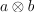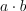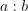/NumPy 1.17

# numpy.tensordot

numpy.tensordot(a, b, axes=2) [source]

Compute tensor dot product along specified axes.

Given two tensors, a and b, and an array_like object containing two array_like objects, (a_axes, b_axes), sum the products of a’s and b’s elements (components) over the axes specified by a_axes and b_axes. The third argument can be a single non-negative integer_like scalar, N; if it is such, then the last N dimensions of a and the first N dimensions of b are summed over.

Parameters: a, b : array_like Tensors to “dot”. axes : int or (2,) array_like integer_like If an int N, sum over the last N axes of a and the first N axes of b in order. The sizes of the corresponding axes must match. (2,) array_like Or, a list of axes to be summed over, first sequence applying to a, second to b. Both elements array_like must be of the same length. output : ndarray The tensor dot product of the input.

See also

#### Notes

Three common use cases are:
• axes = 0 : tensor product• axes = 1 : tensor dot product• axes = 2 : (default) tensor double contractionWhen axes is integer_like, the sequence for evaluation will be: first the -Nth axis in a and 0th axis in b, and the -1th axis in a and Nth axis in b last.

When there is more than one axis to sum over - and they are not the last (first) axes of a (b) - the argument axes should consist of two sequences of the same length, with the first axis to sum over given first in both sequences, the second axis second, and so forth.

#### Examples

A “traditional” example:

>>> a = np.arange(60.).reshape(3,4,5)
>>> b = np.arange(24.).reshape(4,3,2)
>>> c = np.tensordot(a,b, axes=([1,0],[0,1]))
>>> c.shape
(5, 2)
>>> c
array([[4400., 4730.],
[4532., 4874.],
[4664., 5018.],
[4796., 5162.],
[4928., 5306.]])
>>> # A slower but equivalent way of computing the same...
>>> d = np.zeros((5,2))
>>> for i in range(5):
...   for j in range(2):
...     for k in range(3):
...       for n in range(4):
...         d[i,j] += a[k,n,i] * b[n,k,j]
>>> c == d
array([[ True,  True],
[ True,  True],
[ True,  True],
[ True,  True],
[ True,  True]])


An extended example taking advantage of the overloading of + and *:

>>> a = np.array(range(1, 9))
>>> a.shape = (2, 2, 2)
>>> A = np.array(('a', 'b', 'c', 'd'), dtype=object)
>>> A.shape = (2, 2)
>>> a; A
array([[[1, 2],
[3, 4]],
[[5, 6],
[7, 8]]])
array([['a', 'b'],
['c', 'd']], dtype=object)

>>> np.tensordot(a, A) # third argument default is 2 for double-contraction
array(['abbcccdddd', 'aaaaabbbbbbcccccccdddddddd'], dtype=object)

>>> np.tensordot(a, A, 1)
array([[['acc', 'bdd'],
['aaacccc', 'bbbdddd']],
[['aaaaacccccc', 'bbbbbdddddd'],
['aaaaaaacccccccc', 'bbbbbbbdddddddd']]], dtype=object)

>>> np.tensordot(a, A, 0) # tensor product (result too long to incl.)
array([[[[['a', 'b'],
['c', 'd']],
...

>>> np.tensordot(a, A, (0, 1))
array([[['abbbbb', 'cddddd'],
['aabbbbbb', 'ccdddddd']],
[['aaabbbbbbb', 'cccddddddd'],
['aaaabbbbbbbb', 'ccccdddddddd']]], dtype=object)

>>> np.tensordot(a, A, (2, 1))
array([[['abb', 'cdd'],
['aaabbbb', 'cccdddd']],
[['aaaaabbbbbb', 'cccccdddddd'],
['aaaaaaabbbbbbbb', 'cccccccdddddddd']]], dtype=object)

>>> np.tensordot(a, A, ((0, 1), (0, 1)))
array(['abbbcccccddddddd', 'aabbbbccccccdddddddd'], dtype=object)

>>> np.tensordot(a, A, ((2, 1), (1, 0)))
array(['acccbbdddd', 'aaaaacccccccbbbbbbdddddddd'], dtype=object)


© 2005–2019 NumPy Developers
Licensed under the 3-clause BSD License.
https://docs.scipy.org/doc/numpy-1.17.0/reference/generated/numpy.tensordot.html